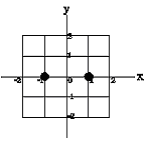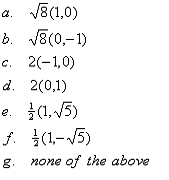Teaching Physics with the Physics Suite Edward F. Redish

E-Field multiple representations

In the figure on the left below is displayed a grid with coordinates measured in meters. On the grid two charges are placed with their positions indicated as black circles. We call the charge at the position (1,0) q1, and the charge at the position (-1,0) q2. In the middle figure below is displayed a set of possible vector directions. On the right is a list of the components of possible E fields. For each of the three cases described below specify: an arrow corresponding to the directions of the E field from the middle figure, and a set of components from the list on the right. Each of your answers should consist of a capital letter and a small letter.Ā

(Note: The values of the charges are chosen to make the messy "4pe0" in Coulomb's law cancel. DON'T PUT IN NUMBERS FIRST!)(a) q1 =0 q2 = 8pe0Ā E field at the point (x,y) = (-1,1) (b) q1 =0 q2 =-8pe0 E field at the point (x,y) = (-1,-1)Ā (c) q1 =16pe0 q2 = -16pe0 E field at the point (x,y) = (0,-1)Ā

Not finding what you wanted? Check the Site Map for more information.